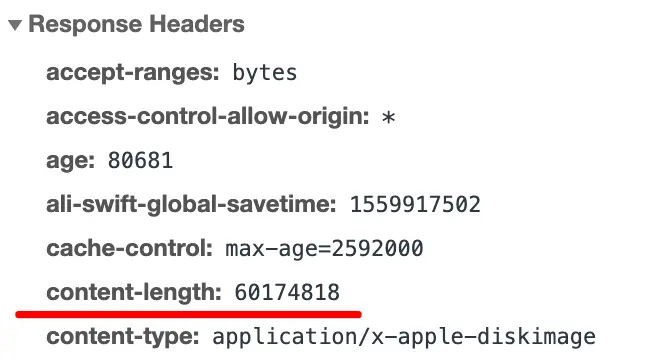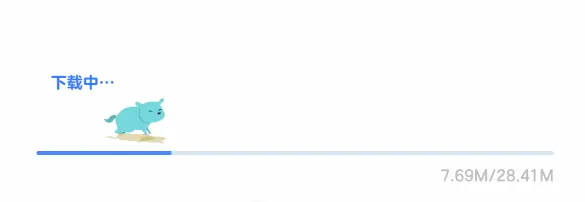# 如何实现一个下载进度条/播放进度条

16,989## 1. 获取下载进度

ajax里面可以拿到下载进度，如下代码所示：

``````  let xhr = new XMLHttpRequest();
// 响应头要有Content-Length
if (event.lengthComputable) {
let percentComplete = event.loaded / event.total;
console.log(percentComplete); // 最后输出1
}
}, false);
xhr.send();````````````let percentComplete = event.loaded / event.total;
let left = containerWidth * percentComplete;
// 狗的位置直接设置translate
dogBox.style.transform = `translateX(\${left}px)`;
// 进度条的位置也是translate，一开始是用translateX(-100%)挪到外面去
currentProgressBar.style.transform = `translateX(\${percentComplete * 100 - 100}%)`;``````

## 3. 加上transform动画

transform动画怎么做呢？方法有很多：jQuery的animate、Web Animation、requestAnimationFrame、CSS动画结合JS控制、其它第三方动画库等等，我比较喜欢用原生Web Animation。

``````// 最快250ms触发一次
function throttle (func, limit = 250) {
let inThrottle = false;
return function() {
const args = arguments;
const context = this;
if (!inThrottle) {
func.apply(context, args);
inThrottle = true;
setTimeout(() => inThrottle = false, limit);
}
}
}

}

``````function onDownloadProgress (event) {
let currentProgressBar = document.querySelector('.current-progress-bar');
let dogBox = document.querySelector('.dog-box');
let containerWidth = document.querySelector('.progress-bar').clientWidth;

if (event.lengthComputable) {
let percentComplete = event.loaded / event.total;
let left = containerWidth * percentComplete;
// 动画时间和节流时间保持一致
const time = 250;
// 获取到当前运动的位移
let lastTransform = window.getComputedStyle(dogBox).transform || 'translateX(0)';
// 使用原生web animation
dogBox.animate({
transform: [lastTransform, `translateX(\${left}px)`]
}, {
easing: 'linear',
fill: 'forwards',
duration: time
});
// 进度条类似，省略
}
}````````````let diffX = (event.loaded - lastMB) / event.total * containerWidth;
// 在原本的基础上再加多一个偏移（且不能超过容器的宽度）
let left = Math.min(containerWidth, containerWidth * percentComplete + diffX);